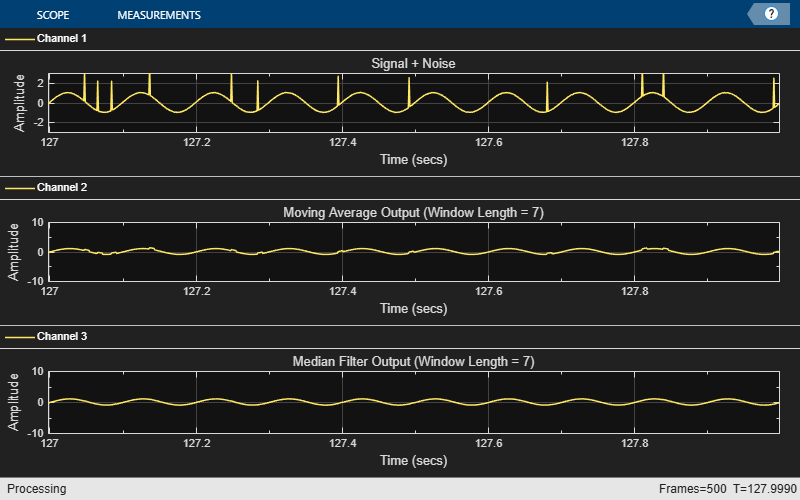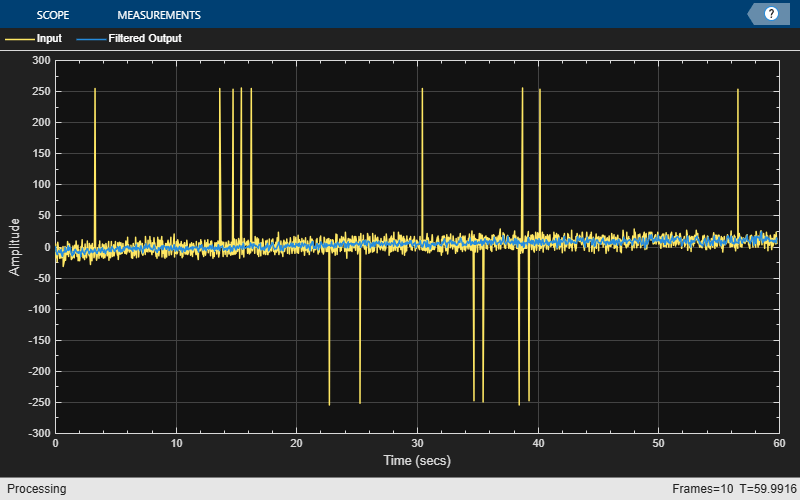# dsp.MedianFilter

Median filter

## Description

The `dsp.MedianFilter` System object™ computes the moving median of the input signal along each channel, independently over time. The object uses the sliding window method to compute the moving median. In this method, a window of specified length is moved over each channel, sample by sample, and the object computes the median of the data in the window. For more details, see Algorithms.

To compute the moving median of the input:

1. Create the `dsp.MedianFilter` object and set its properties.

2. Call the object with arguments, as if it were a function.

## Creation

### Syntax

``medFilt = dsp.MedianFilter``
``medFilt = dsp.MedianFilter(Len)``
``medFilt = dsp.MedianFilter(Name,Value)``

### Description

````medFilt = dsp.MedianFilter` returns a median filter object, `medFilt`, using the default properties.```

example

````medFilt = dsp.MedianFilter(Len)` sets the `WindowLength` property to `Len`.```
````medFilt = dsp.MedianFilter(Name,Value)` specifies the `WindowLength` property using a `Name,Value` pair.Example: movMin = dsp.MedianFilter('WindowLength',5);```

## Properties

expand all

Unless otherwise indicated, properties are nontunable, which means you cannot change their values after calling the object. Objects lock when you call them, and the `release` function unlocks them.

If a property is tunable, you can change its value at any time.

Length of the sliding window in samples, specified as a positive scalar integer.

## Usage

### Syntax

``y = medFilt(x)``

### Description

example

````y = medFilt(x)` computes the moving median of the input signal, `x`, using the sliding window method.```

### Input Arguments

expand all

Data input, specified as a vector or a matrix. If x is a matrix, each column is treated as an independent channel. The moving median is computed along each channel. The object accepts multichannel inputs, that is, m-by-n size inputs, where m ≥ 1, and n > 1. m is the number of samples in each frame (or channel), and n is the number of channels.

The object also accepts variable-size inputs. Once the object is locked, you can change the size of each input channel, but you cannot change the number of channels.

Data Types: `single` | `double`

### Output Arguments

expand all

Filtered signal, returned as a vector or a matrix. The size and data type of the output matches the size and data type of the input.

Data Types: `single` | `double`

## Object Functions

To use an object function, specify the System object as the first input argument. For example, to release system resources of a System object named `obj`, use this syntax:

`release(obj)`

expand all

 `step` Run System object algorithm `release` Release resources and allow changes to System object property values and input characteristics `reset` Reset internal states of System object

## Examples

collapse all

Filter high-frequency noise from a noisy sine wave signal using a median filter. Compare the performance of the median filter with an averaging filter.

Initialization

Set up a `dsp.MedianFilter` object, `medFilt`, and a `dsp.MovingAverage` object, `movavgWin`. These objects use the sliding window method with a window length of 7. Create a time scope for viewing the output.

```Fs = 1000; medFilt = dsp.MedianFilter(7); movavgWin = dsp.MovingAverage(7); scope = timescope('SampleRate',Fs,... 'TimeSpanSource','Property',... 'TimeSpanOverrunAction','Scroll',... 'TimeSpan',1,'ShowGrid',true,... 'YLimits',[-3 3],... 'LayoutDimensions',[3 1],... 'NumInputPorts',3); scope.ActiveDisplay = 1; scope.Title = 'Signal + Noise'; scope.ActiveDisplay = 2; scope.Title = 'Moving Average Output (Window Length = 7)'; scope.ActiveDisplay = 3; scope.Title = 'Median Filter Output (Window Length = 7)'; FrameLength = 256; count = 1; sine = dsp.SineWave('SampleRate',Fs,'Frequency',10,... 'SamplesPerFrame',FrameLength); ```

Filter the Noisy Sine Wave

Generate a noisy sine wave signal with a frequency of 10 Hz. Apply the median filter and the moving average object to the signal. View the output on the time scope.

```for i = 1:500 hfn = 3 * (rand(FrameLength,1) < 0.02); x = sine() + 1e-2 * randn(FrameLength,1) + hfn; y1 = movavgWin(x); y2 = medFilt(x); scope(x,y1,y2); end ```The median filter removes the high-frequency noise more effectively than the moving average object does.

This example shows how to remove the high-frequency outliers from a streaming signal using the `dsp.MedianFilter` System object?.

Use the `dsp.MatFileReader` System object to read the gyroscope MAT file. The gyroscope MAT file contains 3 columns of data, with each column containing 7140 samples. The three columns represent the X-axis, Y-axis, and Z-axis data from the gyroscope motion sensor. Choose a frame size of 714 samples so that each column of the data contains 10 frames. The `dsp.MedianFilter` System object uses a window length of 10. Create a `timescope` object to view the filtered output.

```reader = dsp.MatFileReader('SamplesPerFrame',714,'Filename','LSM9DS1gyroData73.mat',... 'VariableName','data'); medFilt = dsp.MedianFilter(10); scope = timescope('NumInputPorts',1,'SampleRate',119,'YLimits',[-300 300],... 'ChannelNames',{'Input','Filtered Output'},... 'TimeSpanSource','Property','TimeSpan',60,'ShowLegend',true); ```

Filter the gyroscope data using the `dsp.MedianFilter` System object. View the filtered Z-axis data in the time scope.

```for i = 1:10 gyroData = reader(); filteredData = medFilt(gyroData); scope([gyroData(:,3),filteredData(:,3)]); end ```The original data contains several outliers. Zoom in on the data to confirm that the median filter removes all the outliers.

## Algorithms

expand all

 Bodenham, Dean. “Adaptive Filtering and Change Detection for Streaming Data.” PH.D. Thesis. Imperial College, London, 2012.

Watch now